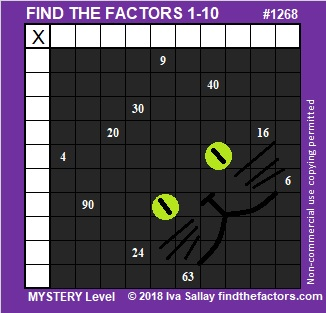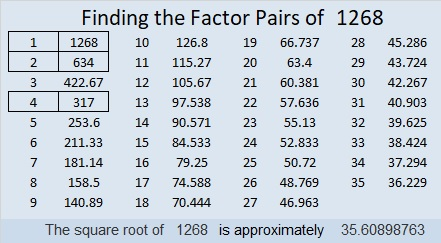1268 Halloween Cat Mystery

Cats can be quite mysterious. They are a favorite pet for many every day, even though suspicious stories abound about them on Halloween. Can you solve the mystery of this cat-like puzzle?Print the puzzles or type the solution in this excel file: 10-factors-1259-1270

Now I’ll share a few facts about the number 1268:

• 1268 is a composite number.
• Prime factorization: 1268 = 2 × 2 × 317, which can be written 1268 = 2² × 317
• The exponents in the prime factorization are 2 and 1. Adding one to each and multiplying we get (2 + 1)(1 + 1) = 3 × 2  = 6. Therefore 1268 has exactly 6 factors.
• Factors of 1268: 1, 2, 4, 317, 634, 1268
• Factor pairs: 1268 = 1 × 1268, 2 × 634, or 4 × 317
• Taking the factor pair with the largest square number factor, we get √1268 = (√4)(√317) = 2√317 ≈ 35.6089928² + 22² = 1268

1268 is the hypotenuse of a Pythagorean triple:
300-1232-1268 calculated from 28² – 22², 2(28)(22), 28² + 22².
It is also 4 times (75-308-317)